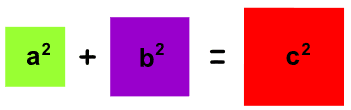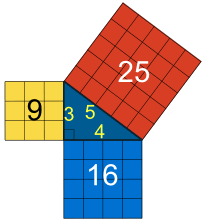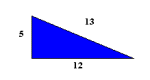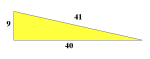# 勾股数

"勾股数" 是一组三个正 整数abc，而且这三个数符合这个规则：

a2 + b2 = c2

32 + 42 = 52

9 + 16 = 25

## 三角形有勾股数的三角形是 直角三角形 （去 勾股定理 了解更多）：注意： c 是三角形的 最长边，叫"斜边" a 和 b 是另外两边。5, 12, 13 9, 40, 4152 + 122 = 132 92 + 402 = 412 25 + 144 = 169 （自己来试试看）

## 最初的几个

 (3,4,5) (5,12,13) (7,24,25) (8,15,17) (9,40,41) (11,60,61) (12,35,37) (13,84,85) (15,112,113) (16,63,65) (17,144,145) (19,180,181) (20,21,29) (20,99,101) (21,220,221) (23,264,265) (24,143,145) (25,312,313) (27,364,365) (28,45,53) (28,195,197) (29,420,421) (31,480,481) (32,255,257) (33,56,65) (33,544,545) (35,612,613) (36,77,85) (36,323,325) (37,684,685) 。。。。。。 还有无限多。。。。。。

62 + 82 = 102

36 + 64 = 100